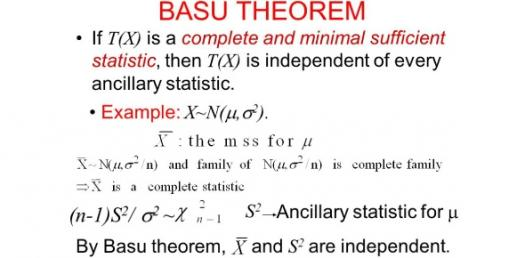# Do You Know Basu's Theorem?

Approved & Edited by ProProfs Editorial Team
The editorial team at ProProfs Quizzes consists of a select group of subject experts, trivia writers, and quiz masters who have authored over 10,000 quizzes taken by more than 100 million users. This team includes our in-house seasoned quiz moderators and subject matter experts. Our editorial experts, spread across the world, are rigorously trained using our comprehensive guidelines to ensure that you receive the highest quality quizzes.
| By Zlatan Aleksic
Z
Zlatan Aleksic
Community Contributor
Quizzes Created: 206 | Total Attempts: 1,002,931
Questions: 10 | Attempts: 140SettingsBasu's theorem gives new insights for statistics. After completing this test you will know how much you are familiar with Basu's theorem! Good luck and have fun whit this quiz!

• 1.

### When was Basu's theorem created?

• A.

1955

• B.

1945

• C.

1935

• D.

1965

A. 1955
Explanation
Basu's theorem was created in 1955.

Rate this question:

• 2.

### What was the name of Basu's theorem creator?

• A.

Subhash

• B.

Debabrata

• C.

Gopinath

• D.

Santosh

B. Debabrata
Explanation
Debabrata is the correct answer because the question asks for the name of the creator of Basu's theorem. Based on the given options, Debabrata is the only name that matches this criteria.

Rate this question:

• 3.

### For what is Basu's theorem often used?

• A.

To resolve statistics

• B.

To measure the value of the statistics

• C.

To prove independence of two statistics

• D.

To test the relationship between two statistical data sets

C. To prove independence of two statistics
Explanation
Basu's theorem is often used to prove the independence of two statistics. This theorem states that if a statistic is ancillary and sufficient, then it is independent of any other statistic that is based on the same sample. In other words, if two statistics are independent, it means that knowing the value of one does not provide any information about the other. Therefore, Basu's theorem is commonly employed to establish the independence between two statistics in statistical analysis.

Rate this question:

• 4.

### With what other theorem independence of two statistics can also be proven?

• A.

Theory of statistics

• B.

Pell's theorem

• C.

Probability theory

• D.

Cochran's theorem

D. Cochran's theorem
Explanation
Cochran's theorem is a statistical theorem that allows us to prove the independence of two statistics. It states that if two statistics are independently distributed under the null hypothesis, then their joint distribution is also independent. This theorem is commonly used in hypothesis testing and regression analysis to verify the independence of different statistical measures. Therefore, Cochran's theorem provides a reliable method to establish the independence of two statistics in the field of statistics.

Rate this question:

• 5.

### Where was Basu's theorem published?

• A.

Sankhya

• B.

Mahabharata - A Modern Retelling

• C.

Sanskrit for Seekers

• D.

A. Sankhya
Explanation
Basu's theorem was published in the journal called Sankhya.

Rate this question:

• 6.

### When was Debabrata Basu born?

• A.

1916

• B.

1924

• C.

1942

• D.

1961

B. 1924
Explanation
Debabrata Basu was born in 1924.

Rate this question:

• 7.

### What is proven in this example (See picture)?

• A.

A is dependent of T

• B.

A is independent of B

• C.

A is independent of T

• D.

B is independent of T

C. A is independent of T
Explanation
The given example shows that A is independent of T. This means that the value or occurrence of A does not depend on the value or occurrence of T. In other words, T does not have any influence or impact on A.

Rate this question:

• 8.

### In whos advanced texts the Basu's theorem makes appearance?

• A.

Lehmann

• B.

• C.

Casella

• D.

All of the above

D. All of the above
Explanation
The Basu's theorem is discussed in advanced texts by Lehmann, Mukhopadhyay, and Casella. This theorem is a fundamental result in statistics that relates the sufficiency and ancillarity of a statistic.

Rate this question:

• 9.

### With which scientist did Basu have intensive discussions in period when his theorem was developed?

• A.

Abraham Wald

• B.

Jerzy Neyman

• C.

Egon Pearson

• D.

Maurice George Kendall

B. Jerzy Neyman
Explanation
Basu had intensive discussions with Jerzy Neyman during the period when his theorem was developed.

Rate this question:

• 10.

### When was Basu elected for Fellow of the American Statistical Association?

• A.

1985

• B.

1989

• C.

1979

• D.

1963

C. 1979
Explanation
Basu was elected as a Fellow of the American Statistical Association in 1979.

Rate this question:

Related TopicsBack to top